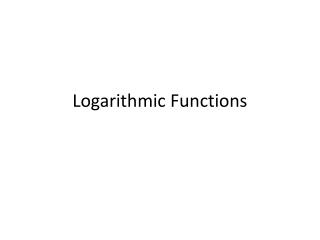DownloadDownload PresentationLogarithmic Functions

Logarithmic Functions

Télécharger la présentationLogarithmic Functions

- - - - - - - - - - - - - - - - - - - - - - - - - - - E N D - - - - - - - - - - - - - - - - - - - - - - - - - - -
Presentation Transcript

1. Logarithmic Functions

2. 1. Write each equation in its equivalent exponential form.(similar to p.434 #4)

3. 2. Write each equation in its equivalent logarithmic form.(similar to p.434 #10)

4. 3. Write each equation in its equivalent logarithmic form.(similar to p.434 #14)

5. 4. Evaluate each expression without using a calculator(similar to p.434 #22)

6. 5. Evaluate each expression without using a calculator(similar to p.434 #28)

7. 6. Evaluate each expression without using a calculator(similar to p.434 #30)

8. 7. Evaluate each expression without using a calculator(similar to p.434 #34)

9. 8. Evaluate each expression without using a calculator(similar to p.434 #38)

10. 9. Evaluate each expression without using a calculator(similar to p.434 #42)

11. 10. The graph of an exponential function is given. Select the function for each graph from the following options: (similar to p.434 #47-52)

12. 11. Describe the transformation and graph the function(similar to p.435 #54)

13. 12. Describe the transformation and graph the function(similar to p.435 #62)

14. 13. Describe the transformation and graph the function(similar to p.435 #68)

15. 16. Evaluate or simplify each expression without using a calculator(similar to p.435 #82)

16. 17. Evaluate or simplify each expression without using a calculator(similar to p.435 #92)

17. 18. Evaluate or simplify each expression without using a calculator(similar to p.435 #98)

18. 19. Evaluate or simplify each expression without using a calculator(similar to p.435 #100)